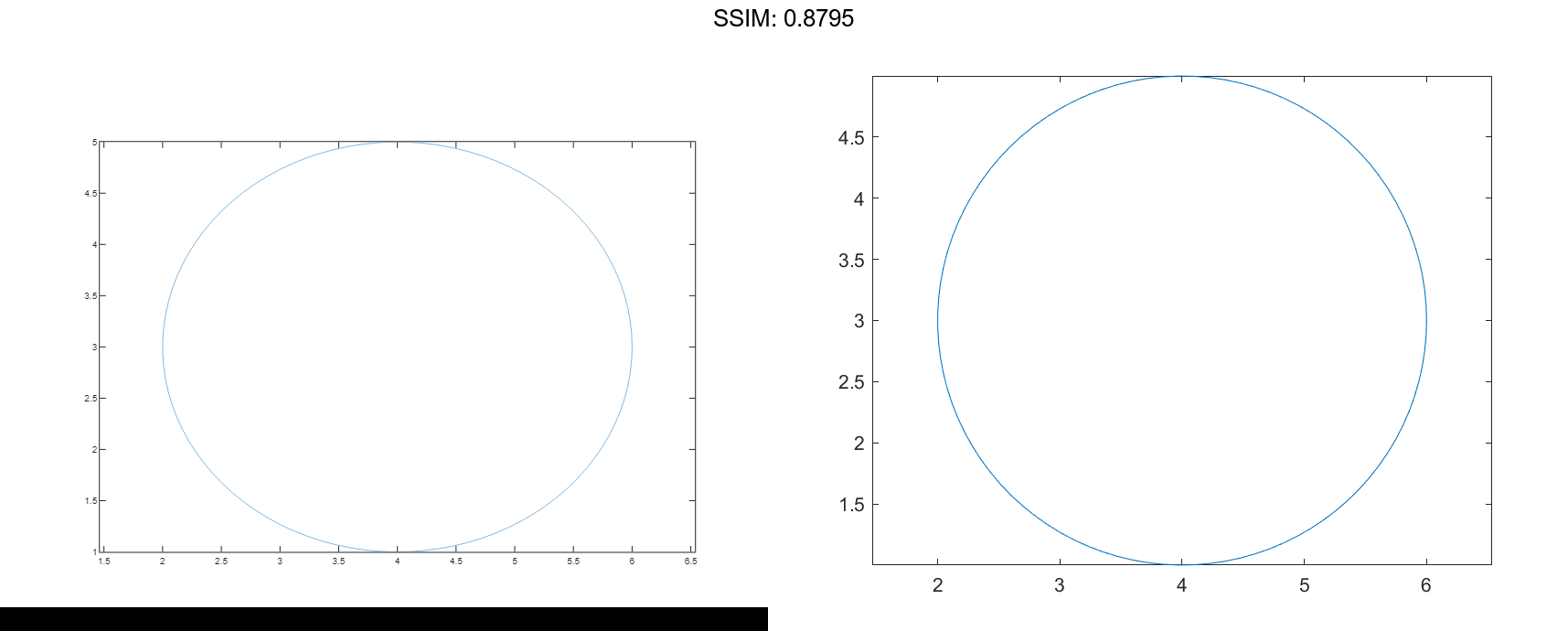# Line Charts in MATLAB®

How to make Line Charts plots in MATLAB® with Plotly.

## Create Line Plot

Create x as a vector of linearly spaced values between 0 and 2π. Use an increment of π/100 between the values. Create y as sine values of x. Create a line plot of the data.

x = 0:pi/100:2*pi;
y = sin(x);
plot(x,y)

fig2plotly()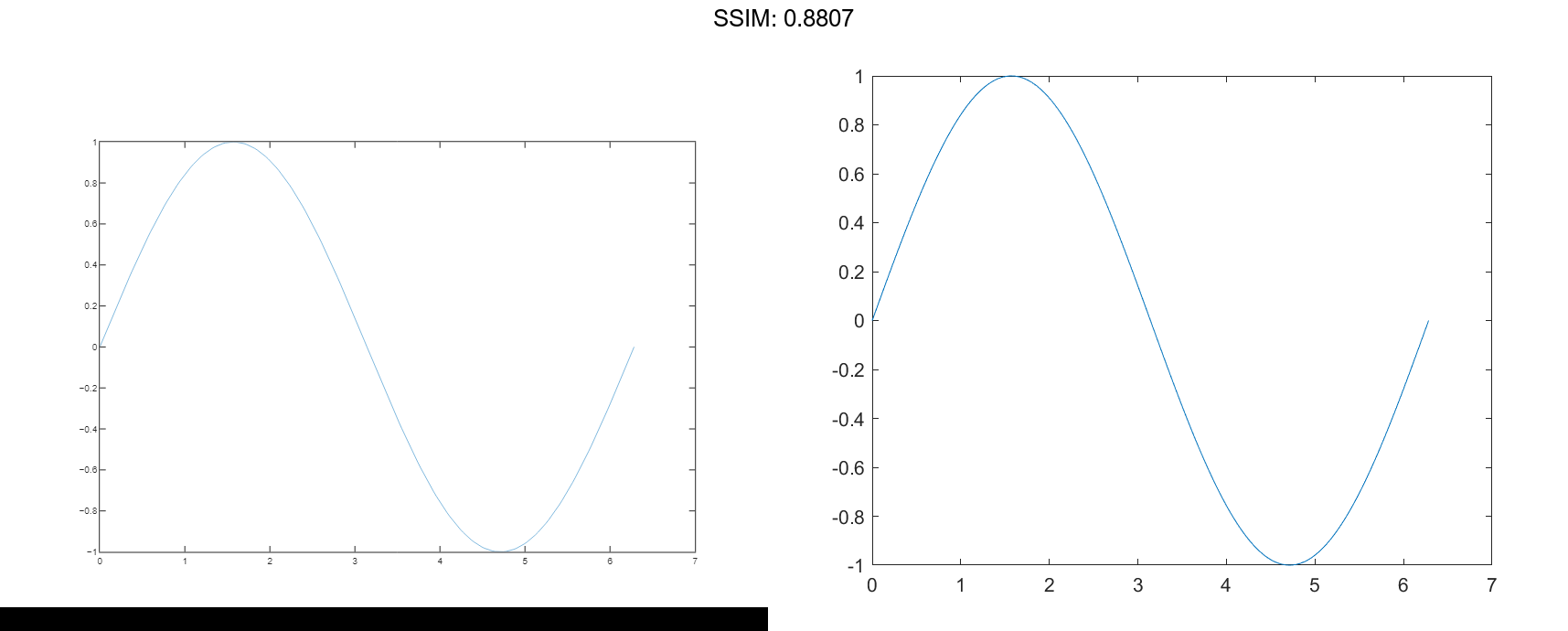## Plot Multiple Lines

Define x as 100 linearly spaced values between -2π and 2π. Define y1 and y2 as sine and cosine values of x. Create a line plot of both sets of data.

x = linspace(-2*pi,2*pi);
y1 = sin(x);
y2 = cos(x);

figure
plot(x,y1,x,y2)

fig2plotly()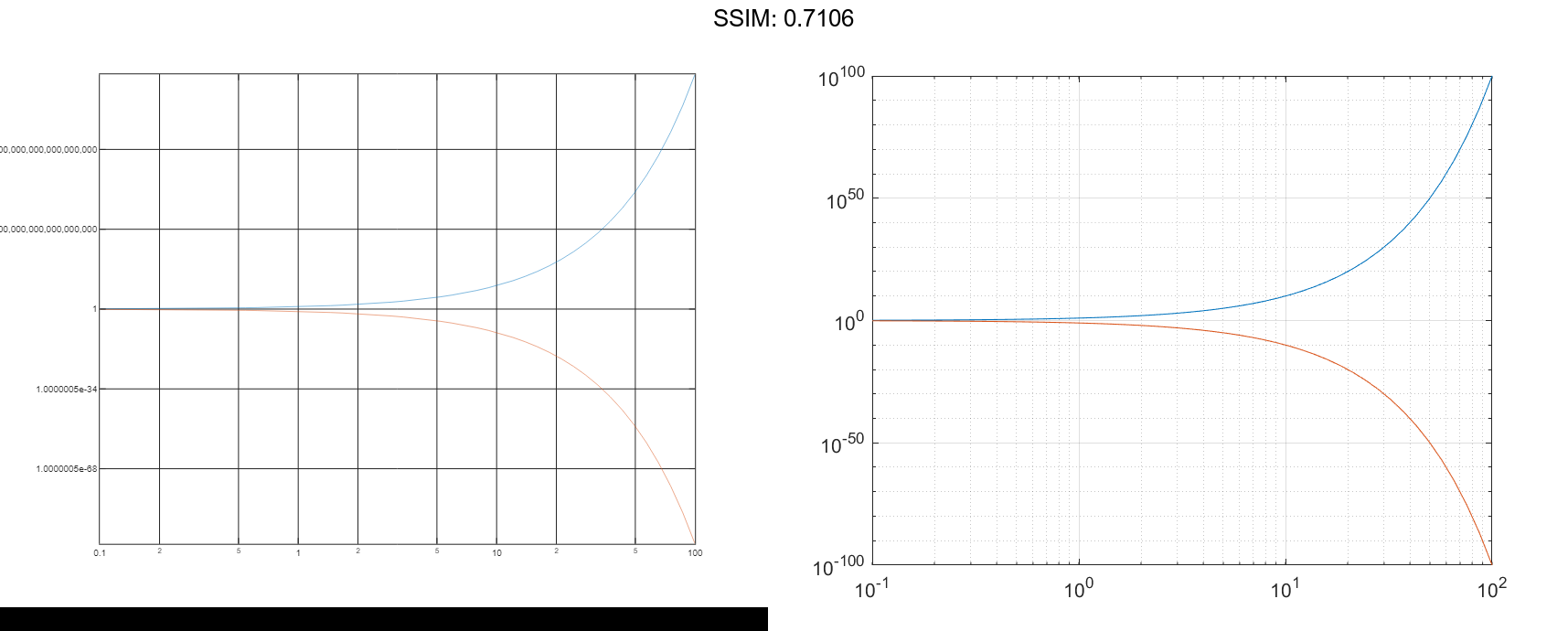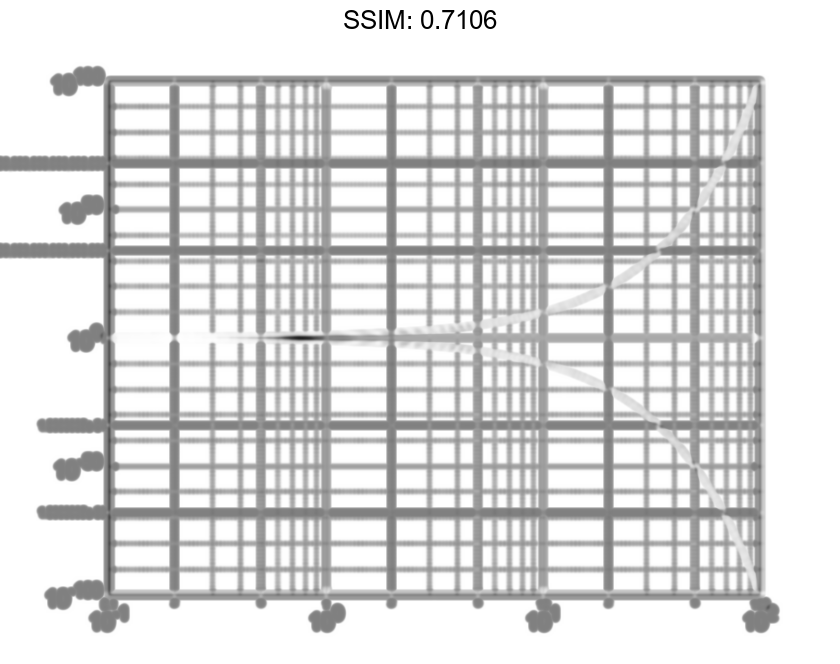## Create Line Plot From Matrix

Define Y as the 4-by-4 matrix returned by the magic function.

Y = magic(4)

Y = 4×4

16     2     3    13
5    11    10     8
9     7     6    12
4    14    15     1



Create a 2-D line plot of Y. MATLAB® plots each matrix column as a separate line.

figure
plot(Y)

fig2plotly()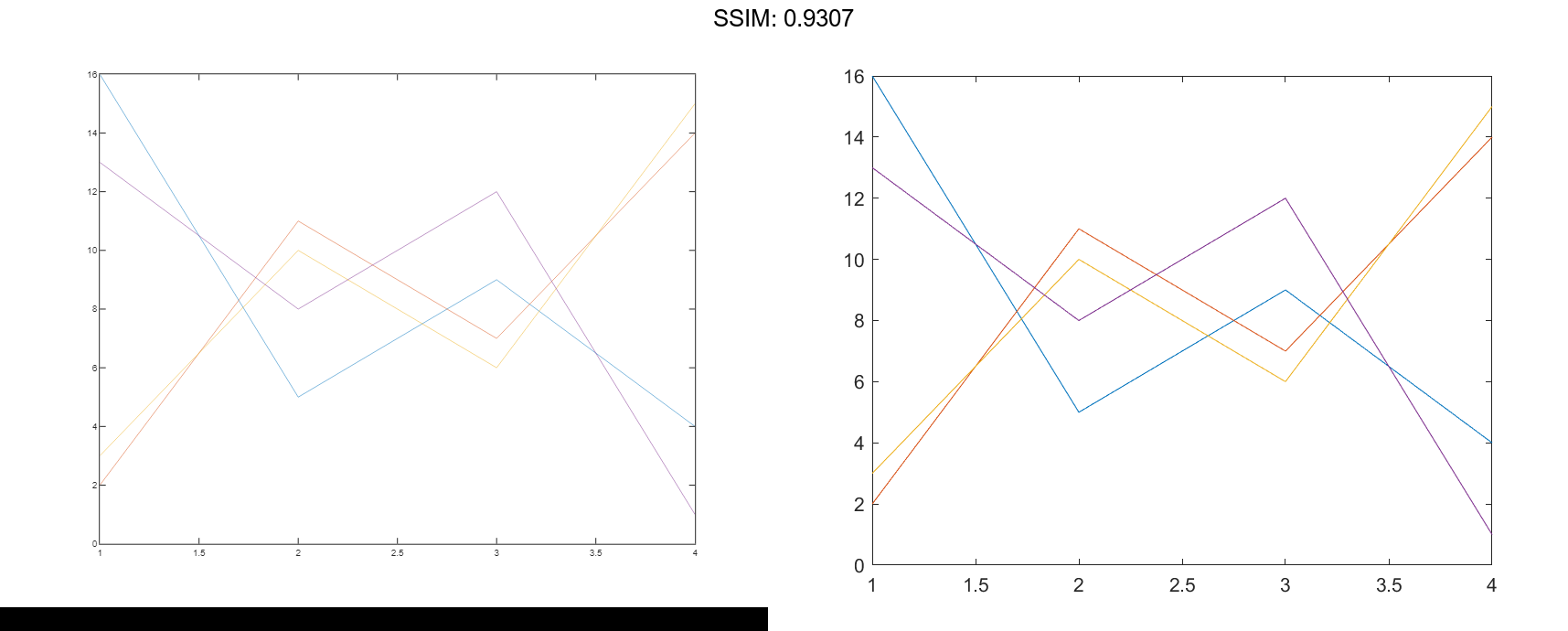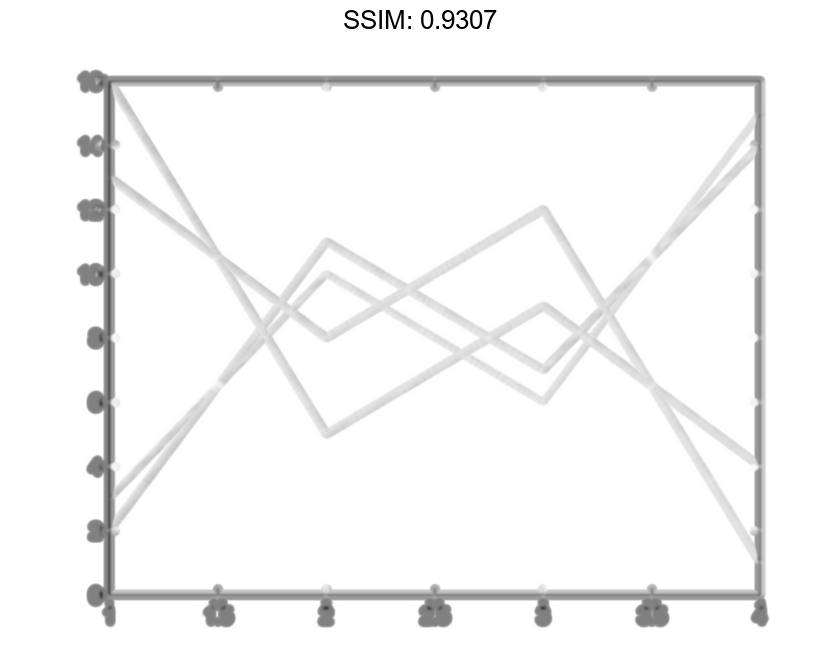## Specify Line Style

Plot three sine curves with a small phase shift between each line. Use the default line style for the first line. Specify a dashed line style for the second line and a dotted line style for the third line.

x = 0:pi/100:2*pi;
y1 = sin(x);
y2 = sin(x-0.25);
y3 = sin(x-0.5);

figure
plot(x,y1,x,y2,'--',x,y3,':')

fig2plotly()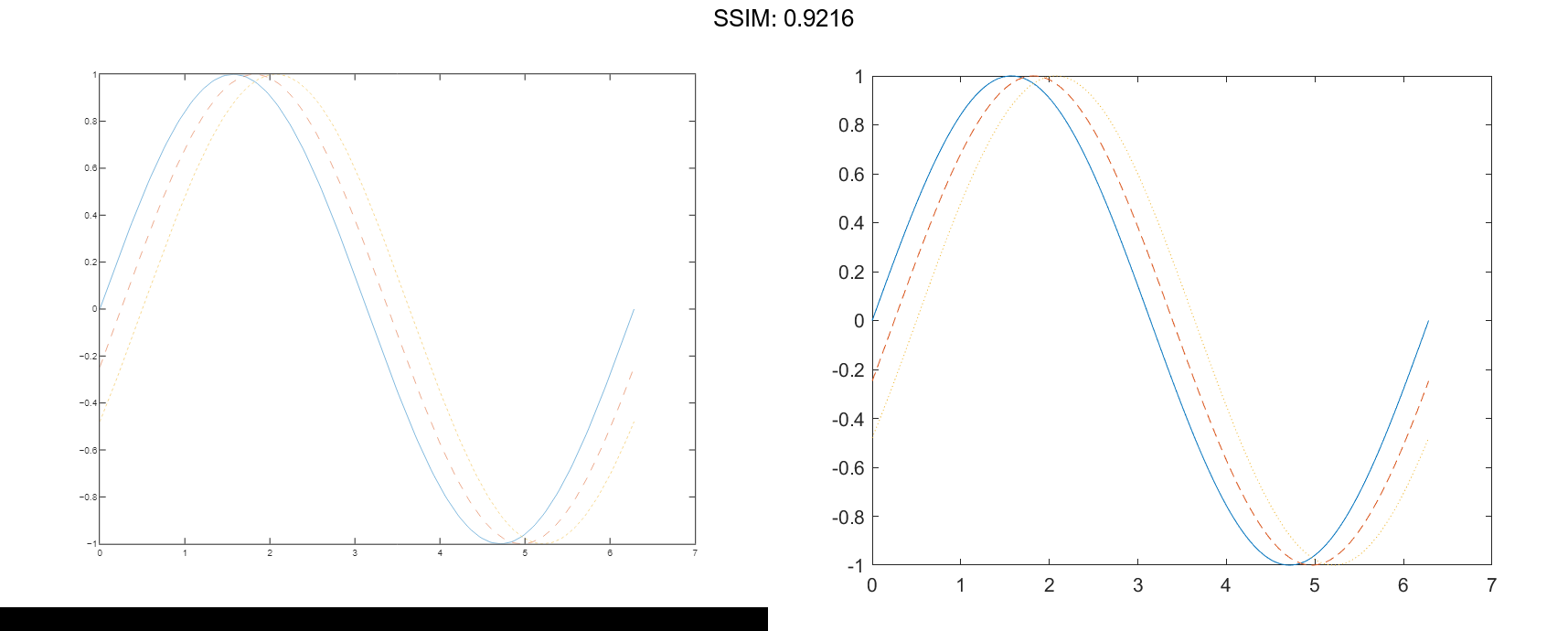MATLAB® cycles the line color through the default color order.

## Specify Line Style, Color, and Marker

Plot three sine curves with a small phase shift between each line. Use a green line with no markers for the first sine curve. Use a blue dashed line with circle markers for the second sine curve. Use only cyan star markers for the third sine curve.

x = 0:pi/10:2*pi;
y1 = sin(x);
y2 = sin(x-0.25);
y3 = sin(x-0.5);

figure
plot(x,y1,'g',x,y2,'b--o',x,y3,'c*')

fig2plotly()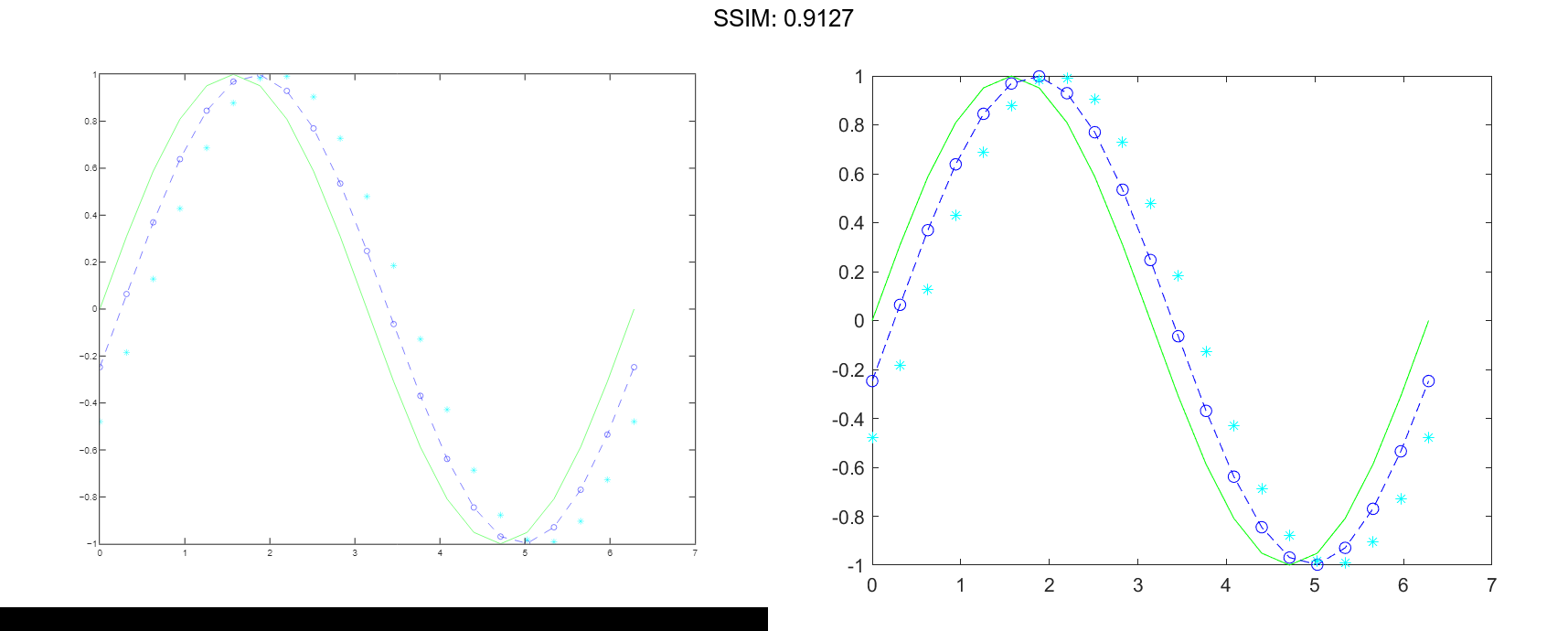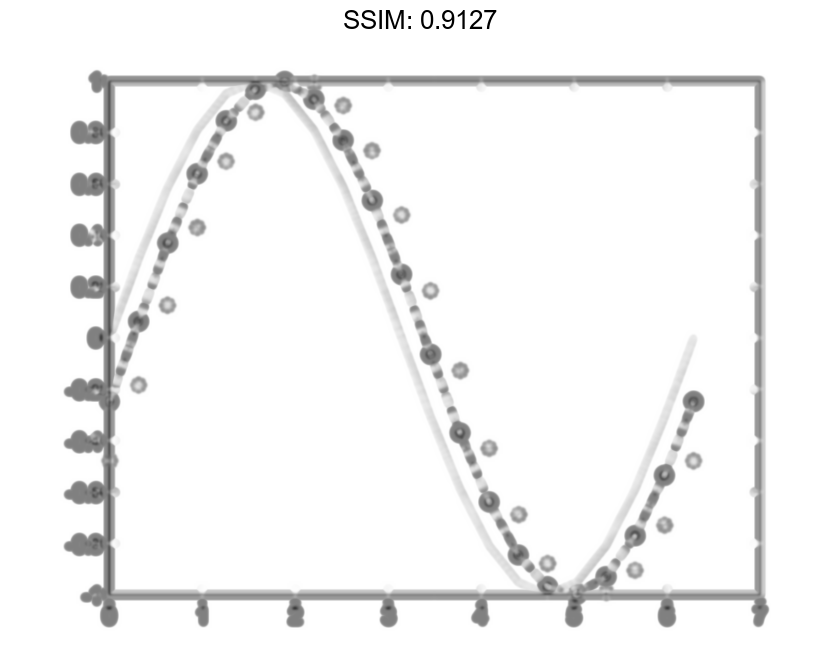## Display Markers at Specific Data Points

Create a line plot and display markers at every fifth data point by specifying a marker symbol and setting the MarkerIndices property as a name-value pair.

x = linspace(0,10);
y = sin(x);
plot(x,y,'-o','MarkerIndices',1:5:length(y))

fig2plotly()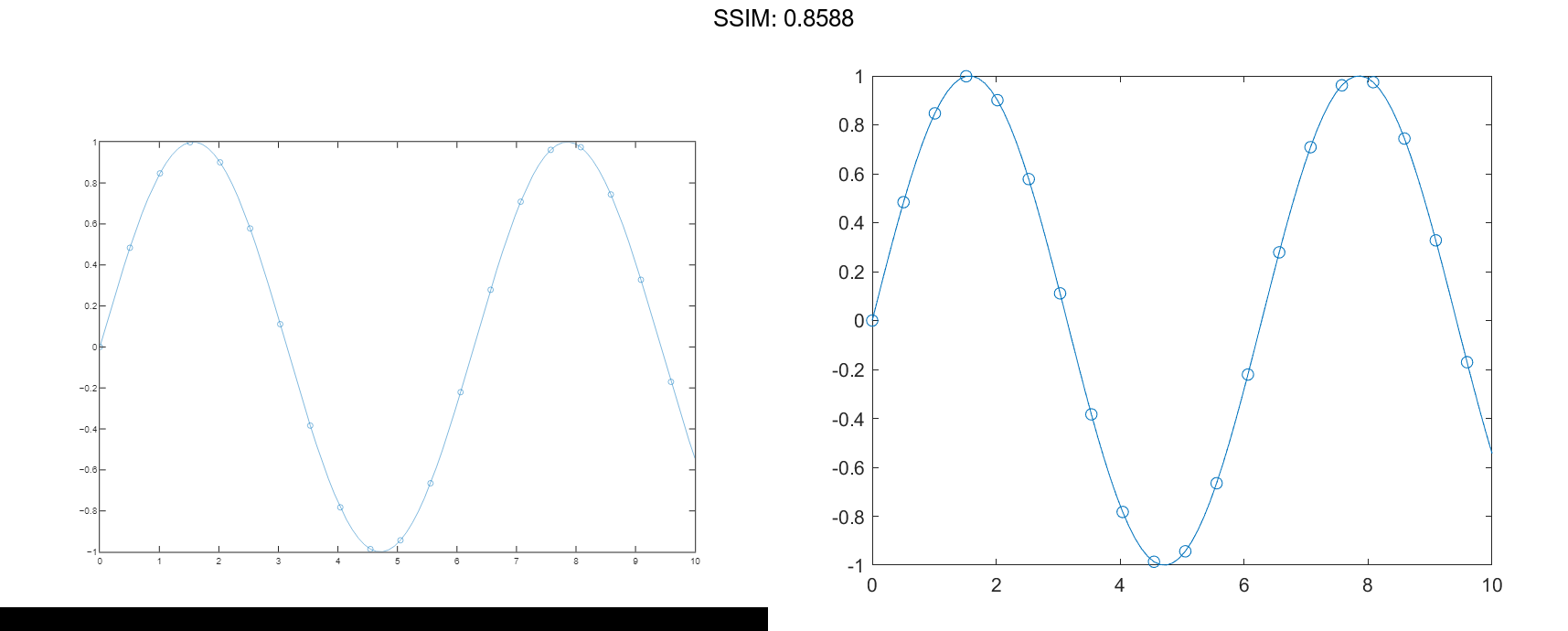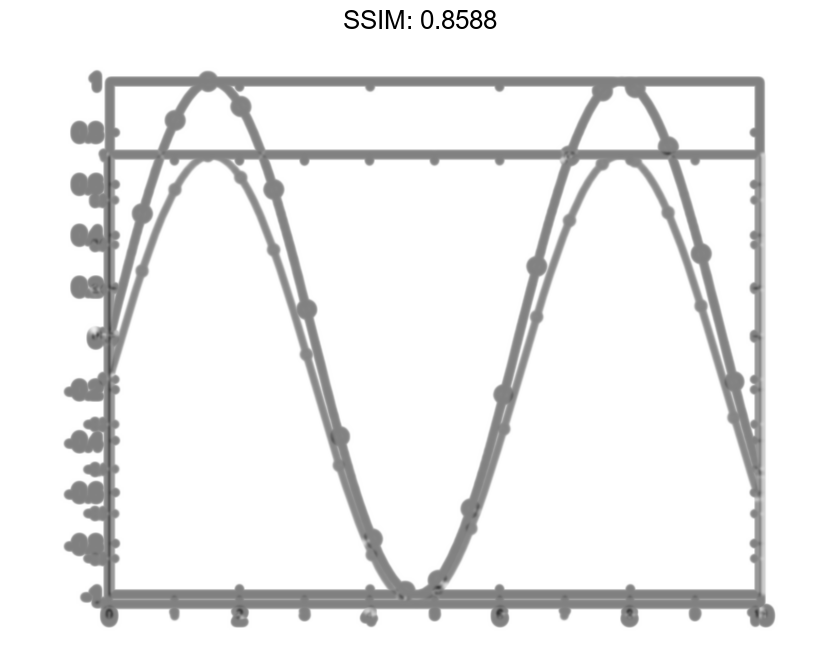## Specify Line Width, Marker Size, and Marker Color

Create a line plot and use the LineSpec option to specify a dashed green line with square markers. Use Name,Value pairs to specify the line width, marker size, and marker colors. Set the marker edge color to blue and set the marker face color using an RGB color value.

x = -pi:pi/10:pi;
y = tan(sin(x)) - sin(tan(x));

figure
plot(x,y,'--gs',...
'LineWidth',2,...
'MarkerSize',10,...
'MarkerEdgeColor','b',...
'MarkerFaceColor',[0.5,0.5,0.5])

fig2plotly()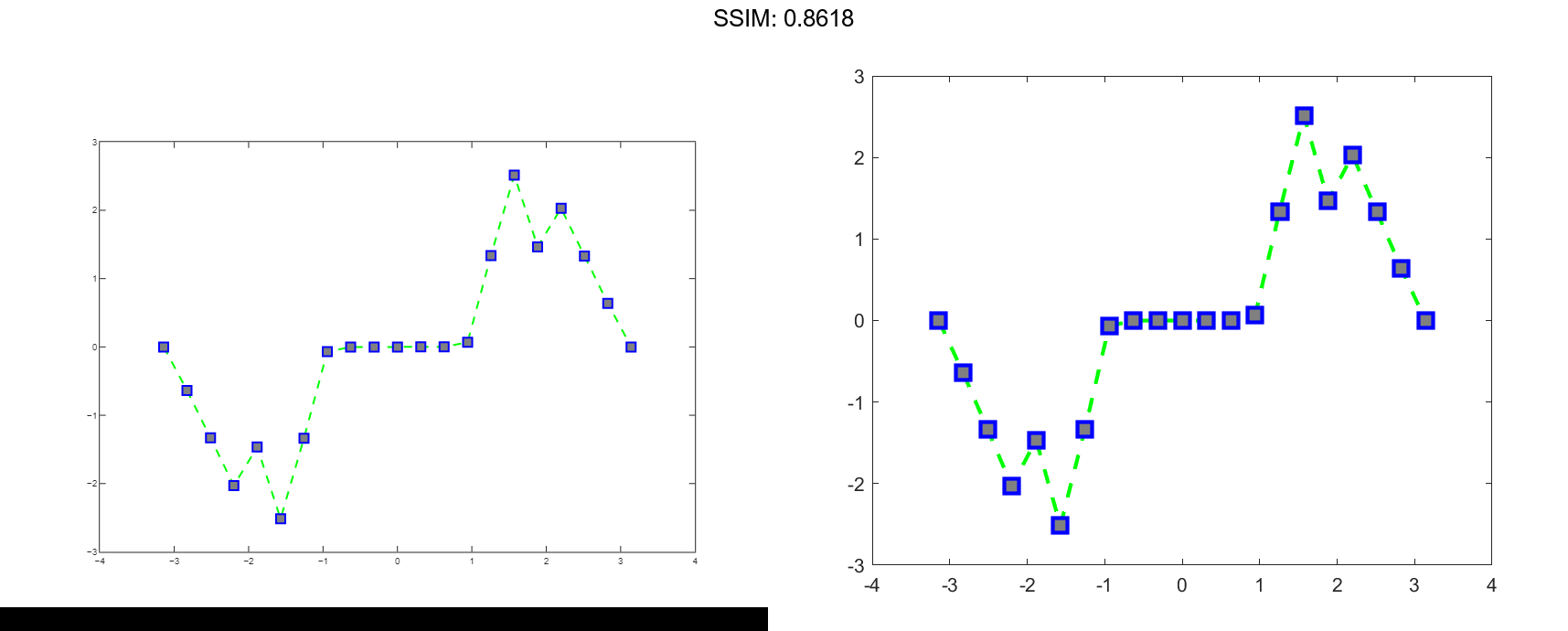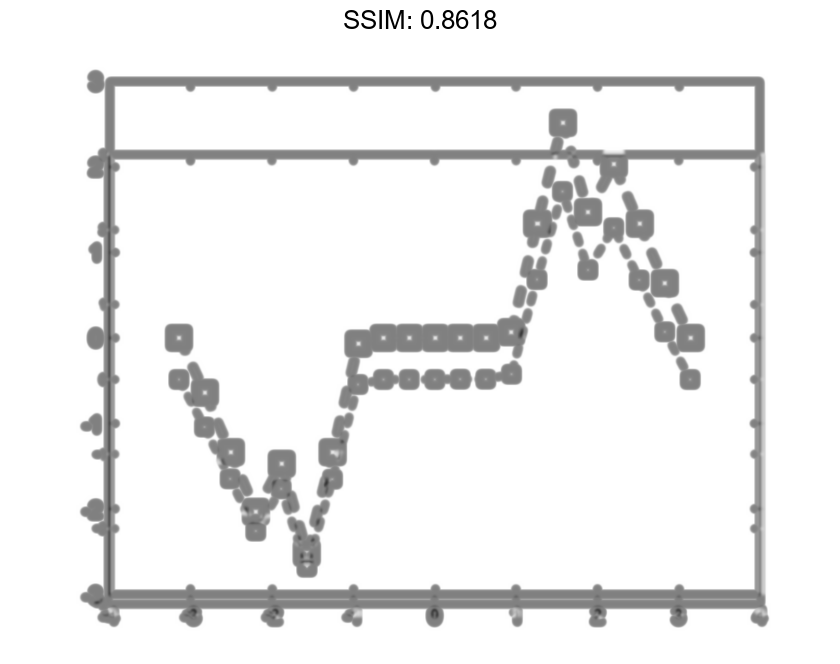## Add Title and Axis Labels

Use the linspace function to define x as a vector of 150 values between 0 and 10. Define y as cosine values of x.

x = linspace(0,10,150);
y = cos(5*x);


Create a 2-D line plot of the cosine curve. Change the line color to a shade of blue-green using an RGB color value. Add a title and axis labels to the graph using the title, xlabel, and ylabel functions.

figure
plot(x,y,'Color',[0,0.7,0.9])

title('2-D Line Plot')
xlabel('x')
ylabel('cos(5x)')

fig2plotly()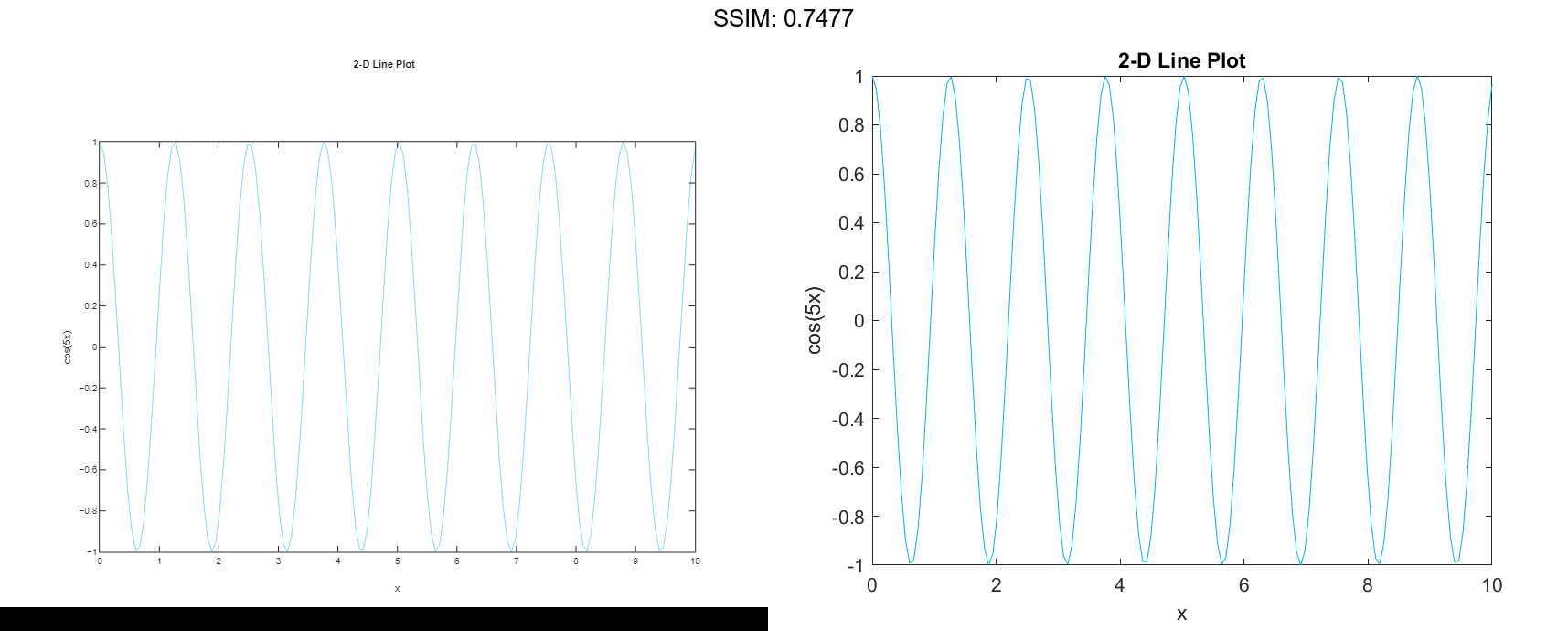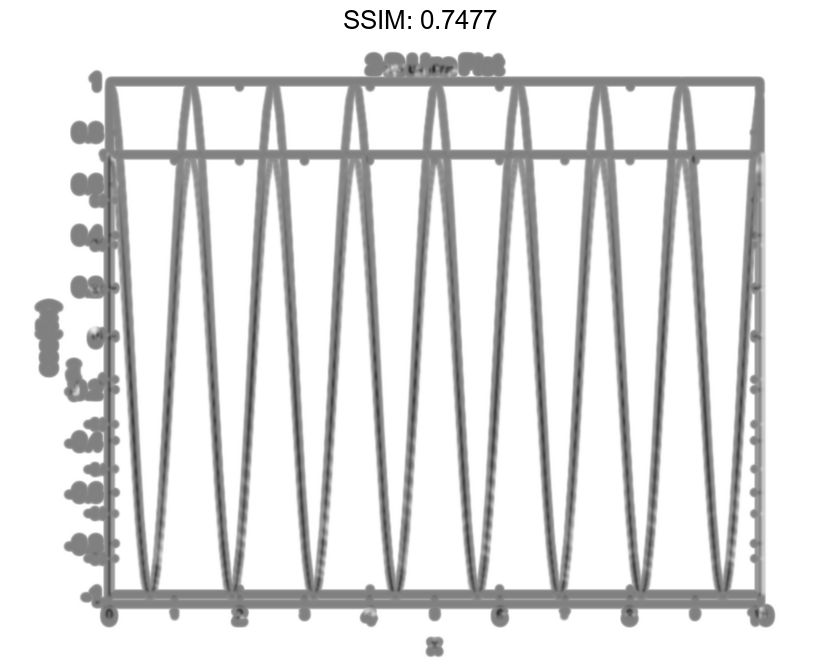## Plot Durations and Specify Tick Format

Define t as seven linearly spaced duration values between 0 and 3 minutes. Plot random data and specify the format of the duration tick marks using the 'DurationTickFormat' name-value pair argument.

t = 0:seconds(30):minutes(3);
y = rand(1,7);

plot(t,y,'DurationTickFormat','mm:ss')

fig2plotly()## Specify Axes for Line Plot

Starting in R2019b, you can display a tiling of plots using the tiledlayout and nexttile functions. Call the tiledlayout function to create a 2-by-1 tiled chart layout. Call the nexttile function to create an axes object and return the object as ax1. Create the top plot by passing ax1 to the plot function. Add a title and y-axis label to the plot by passing the axes to the title and ylabel functions. Repeat the process to create the bottom plot.

% Create data and 2-by-1 tiled chart layout
x = linspace(0,3);
y1 = sin(5*x);
y2 = sin(15*x);
tiledlayout(2,1)

% Top plot
ax1 = nexttile;
plot(ax1,x,y1)
title(ax1,'Top Plot')
ylabel(ax1,'sin(5x)')

% Bottom plot
ax2 = nexttile;
plot(ax2,x,y2)
title(ax2,'Bottom Plot')
ylabel(ax2,'sin(15x)')

fig2plotly()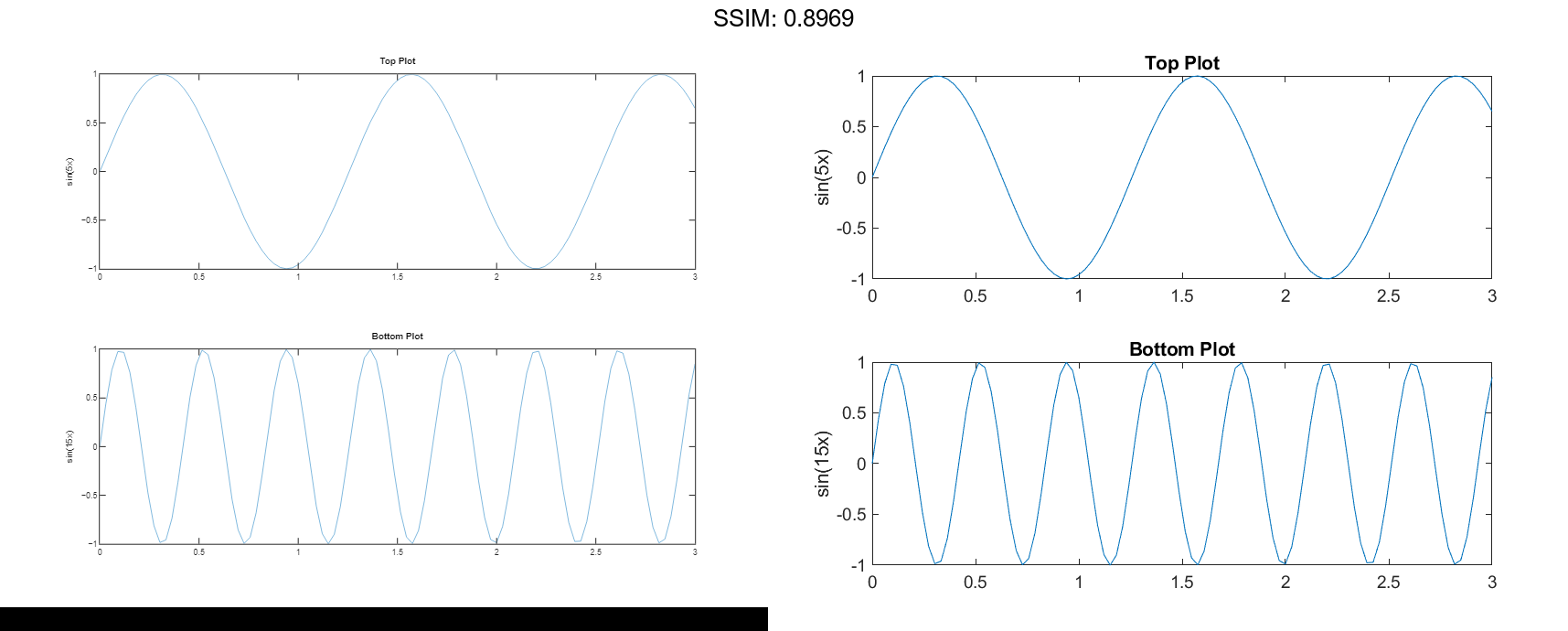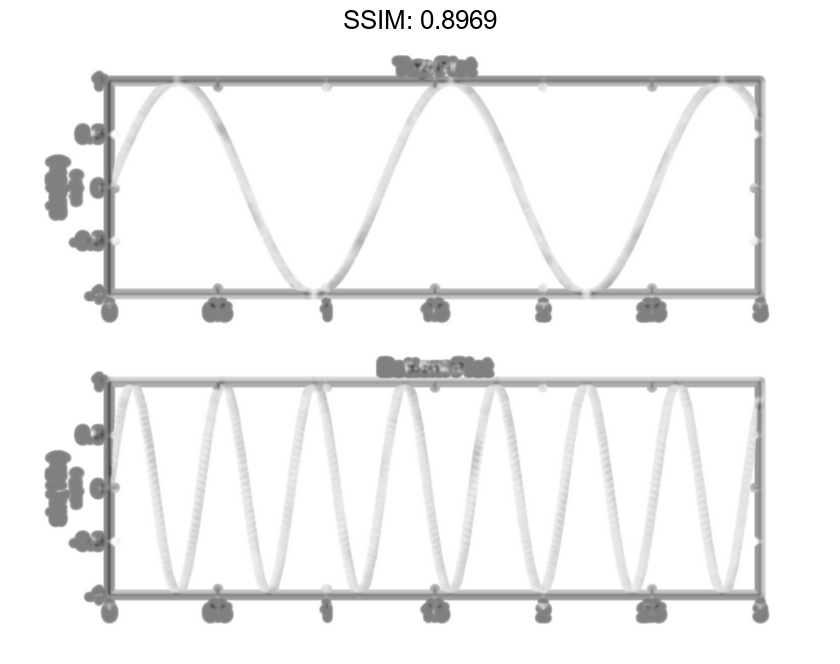## Modify Lines After Creation

Define x as 100 linearly spaced values between -2π and 2π. Define y1 and y2 as sine and cosine values of x. Create a line plot of both sets of data and return the two chart lines in p.

x = linspace(-2*pi,2*pi);
y1 = sin(x);
y2 = cos(x);
p = plot(x,y1,x,y2);

fig2plotly()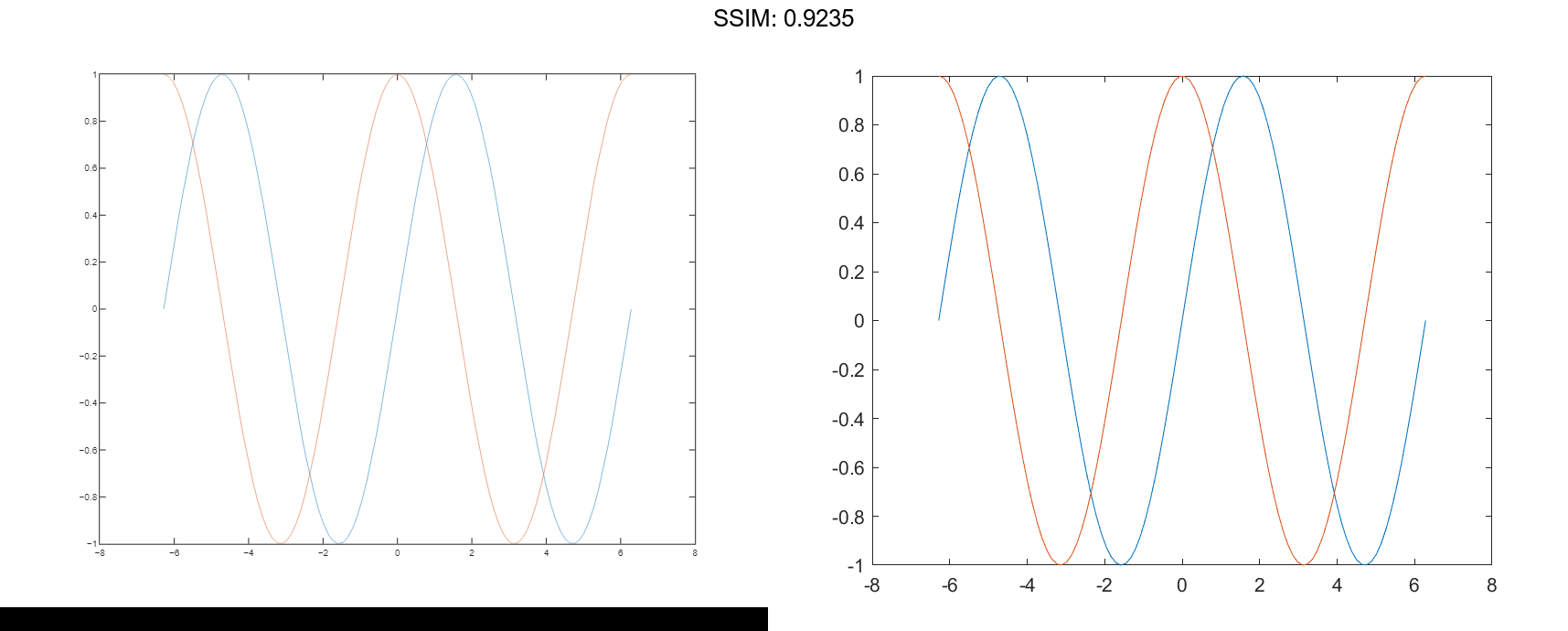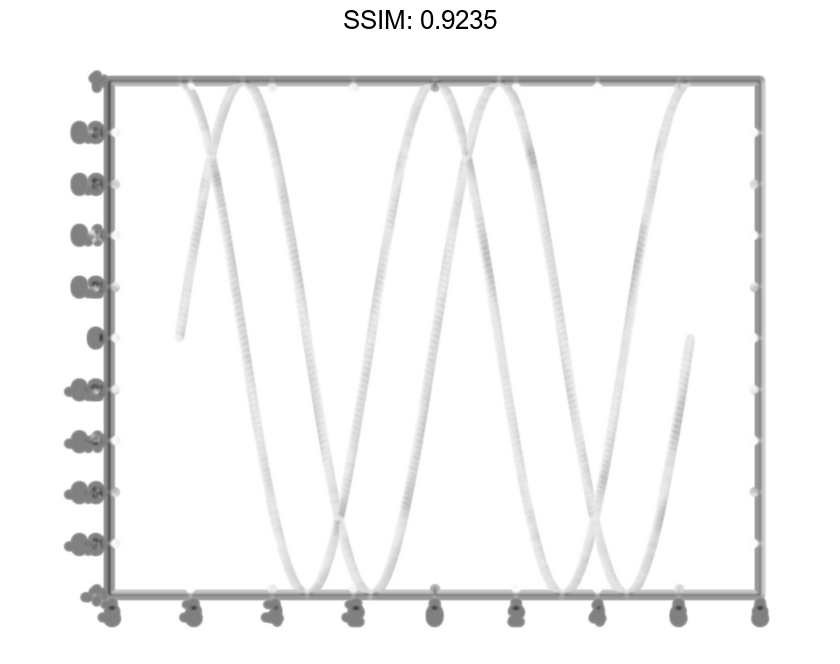Change the line width of the first line to 2. Add star markers to the second line. Use dot notation to set properties.

p(1).LineWidth = 2;
p(2).Marker = '*';

fig2plotly()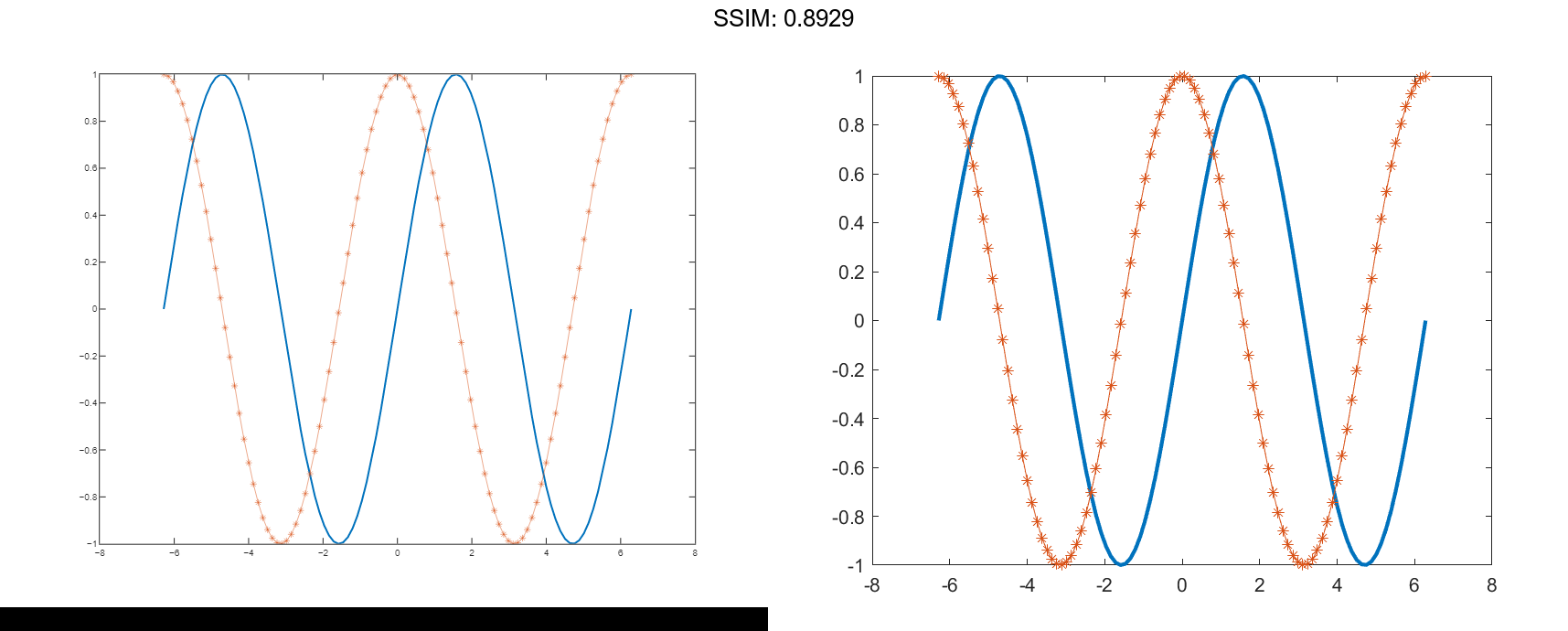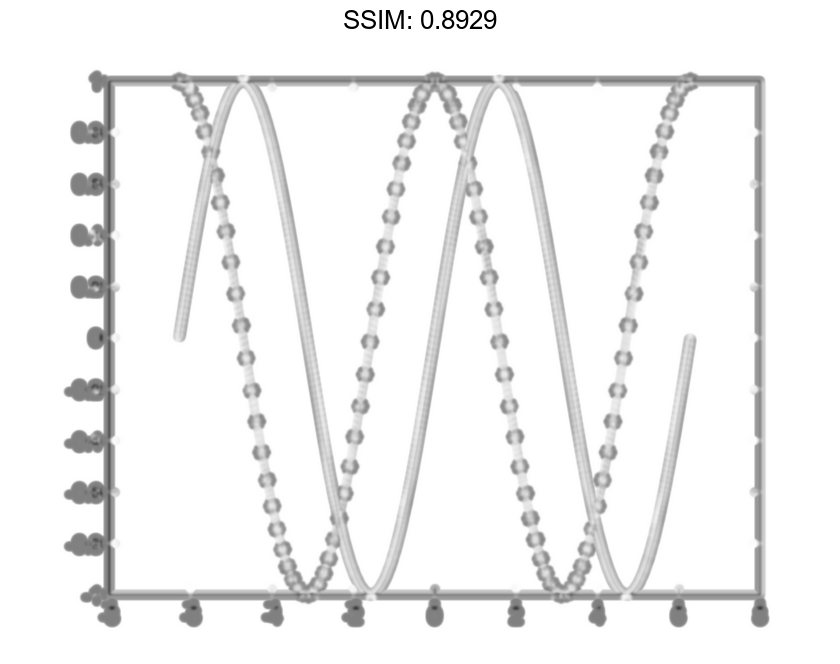## Plot Circle

Plot a circle centered at the point (4,3) with a radius equal to 2. Use axis equal to use equal data units along each coordinate direction.

r = 2;
xc = 4;
yc = 3;

theta = linspace(0,2*pi);
x = r*cos(theta) + xc;
y = r*sin(theta) + yc;
plot(x,y)
axis equal

fig2plotly()